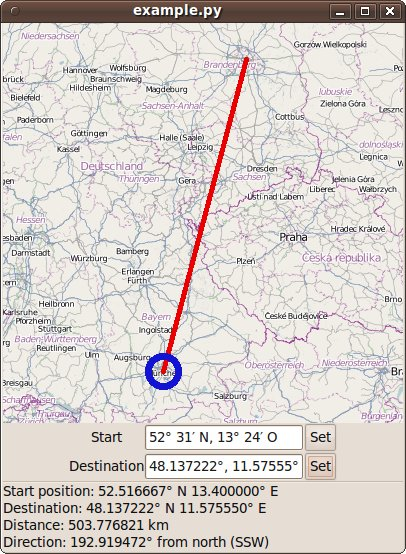home# geo.py

geo.py is a small python module with no external dependencies that can do some simple vector calculations with geographic coordinates, primarily give the direction and distance from a start point to a destination.

Moreover, it contains a parser for geographic coordinates that aims to accept all possible input formats of simple geographic coordinates.

## Examples

### Example application

example.py is an example application that shows the capabilities of geo.py (see screenshot). The map is drawn by python-osmgpsmap.

### get distance between two points on a sphere

``````#!/usr/bin/env python

import geo

berlin=geo.xyz(52.518611,13.408056)
munich=geo.xyz(48.137222,11.575556)

print geo.distance(berlin,munich)
``````

This will print 504131.727339, the distance from Berlin to Munich in meters.

### determine travelling direction for a compass

``````#!/usr/bin/env python

import geo

berlin=geo.xyz(52.518611,13.408056)
munich=geo.xyz(48.137222,11.575556)

print geo.great_circle_angle(munich,berlin,geo.magnetic_northpole)
``````

This will print 204.239820331, the direction in degrees you would have to adjust your compass to when you want to go from Berlin to Munich if there were no declination.

This does NOT work, because there are strong irregularities of the magnetic field of the earth, that's why the compass needle does not point to the magnetic northpole on every point of the earth. A database containing the magnetic declination, the angle between local magnetic field and true north, for every point on the earth is necessary to compensate this.

There is a python module called geomag that can compensate this effect using the IGRF Model:

``````#!/usr/bin/env python

import geo
import geomag

berlin_lat = 52.518611
berlin_lon = 13.408056

berlin=geo.xyz(berlin_lat,berlin_lon)
munich=geo.xyz(48.137222,11.575556)

true_north = geo.great_circle_angle(munich,berlin,geo.geographic_northpole)
``````

This will print 192.976306521, if you adjust your compass to this angle you will actually get to Munich.

### get cardinal directions from angle

``````#!/usr/bin/env python

import geo

print geo.direction_name(337.)
``````

This will print "NNW", the cardinal direction corresponding to an angle of 337° from north.

### Parse a position

``````#!/usr/bin/env python
# -*- coding: utf-8 -*-

import geo

print geo.parse_position("48° 8′ 0″ N, 11° 34′ 0″ E")
print geo.parse_position("48° 8′ 0″ N, -11° 34' 0'' W")
print geo.parse_position(" 11° 34′ O 48° 8´ 0″ N")
print geo.parse_position(" +1134.0 O 48° 8` 0″ N")
print geo.parse_position("48° 8′ 0″  11° 34′ ")
print geo.parse_position("48.133333333333333; +11.566666666666666")
``````

This will print six times the same (latitude,longitude) tuple (48.133333333333333, 11.566666666666666), as all these strings represent the same position.